## Bias In Recommender System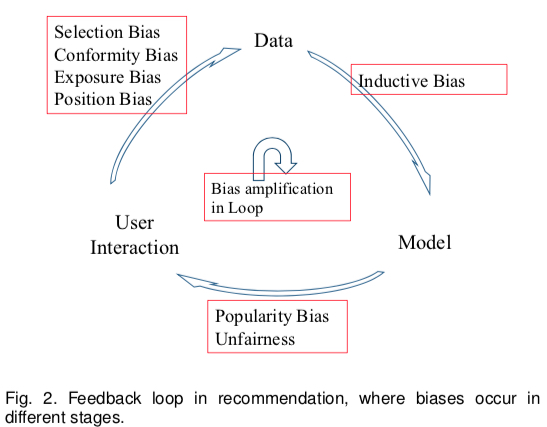• User->Data: 产生训练数据的过程，也是 Bias 的最主要来源

• Position Bias(implicit)：用户更倾向于和位置靠前的物品进行交互
• Exposure/Observation Bias(implicit)：带标签的数据都是曝光过的，未曝光的数据无法确定其标签
• Selection Bias(explicit)：用户倾向于给自己喜欢或者不喜欢的物品进行打分(导致 exposure bias 的一个重要原因，不少 paper 将这个 bias 也当做 exposure bias)
• Conformity Bias(explicit)：用户打分的分数倾向于和群体观点保持一致
• Data->Model: 利用数据训练出模型的过程

• Inductive Bias: 指的是模型为了泛化性而做出的一些 assumption，如 Occam’s razor，SVM 假设线性可分的 assumption 等，这个 bias 没有带来显示的缺陷
• Model->User Interaction: 指的是模型预估的过程

• Popularity Bias：指的是长尾效应，热门物品会得到更高的曝光概率，因为模型会更倾向于推荐这些物品（unbalanced training data 引起）
• Unfairness: 一些预估结果带有性别歧视、种族歧视等（unbalanced training data 引起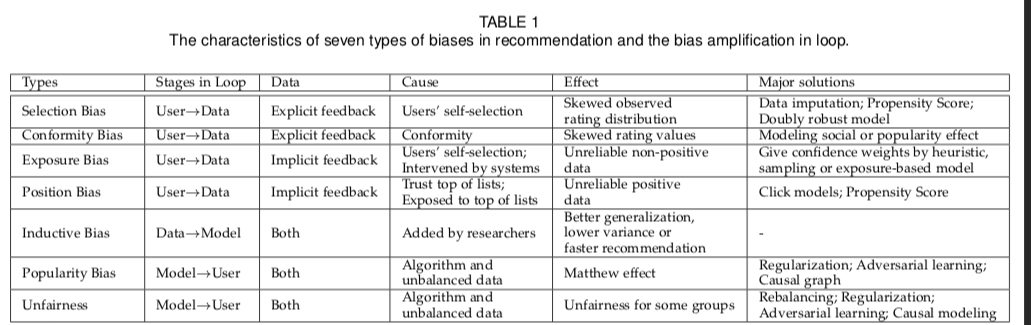## Solutions to Exposure Bias

exposure bias 也被称为 Sample Selection Bias(SSB), 本质上是一个 training 和 serving 不一致的问题。这个问题往往是由于具体业务场景的限制，导致 training data 中的样本只是其 serving 时的很小一部分，因为其他的样本没被曝光/点击，导致了无法得到其 label。

(1) Data Augmentation：这个是最朴素的想法，就是尽可能将那些没进入训练数据集的样本利用上，因此不少研究也是给 unobserved/unclicked 的样本打上一个相对准确的 label；而这里面也主要分为下面三类方法

• 最粗暴的是把所有未曝光的样本当做 negative sample，然后基于不同策略给这些样本不同的权重
• 训练一个 imputation model，然后通过 imputation model 来预估未曝光样本的 label

(2) IPS(Inverse Propensity Score): 这个方法的假设是样本被曝光或点击服一个伯努利分布，然后从概率论推导出：只要给每个曝光的样本加权(权重即为 inverse propensity score)，最终在曝光的样本上求得的期望等于在全量样本上的期望；实际上，这个方法的思想就是 importance sampling

(3) Domain Adaption: 类似 transfer learning 的思想，将曝光/点击的样本视为 source domain，全部样本视为 target domain；通过 domain adaption 的一些方法去进行 debias

### Data Augmentation

#### all nagative with confidence

• Heuristic 尝试将 user activity level、user preference、item popularity、user-item feature similarity 等作为 (user, item) pair 的 confidence, 其思想都是认为用户活跃度越高、商品越热门、用户-商品匹配度越高，该样本的可信度(confidence) 越高；但是这种方法往往可行性不够高，因为这个 confidence 实际的值是比较难获取的，其量纲以及需要对庞大的数据集都生成这个 confidence，难度是很大的。

• Sampling：TODO

• Exposure-based mode：TODO

#### imputation model

training；这个方法的弊端也很明显，就是生成的 imputed label 缺少绝对的 ground truth 来衡量其效果，实际上，这个是所有直接为样本生成 label 的方法的弊端。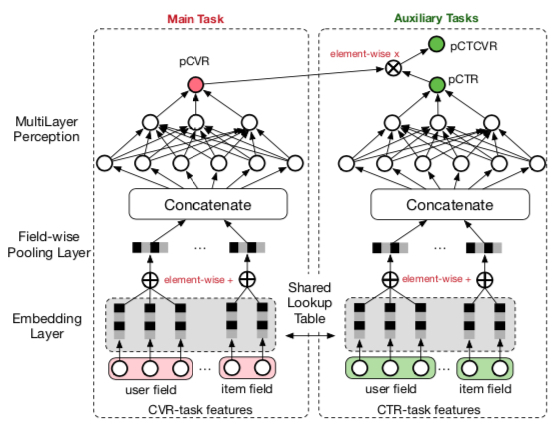\begin{align} L(\theta_{ctr},\theta_{cvr}) &= \sum_{i=1}^{N}l(y_i,f(x_i;\theta_{ctr})) \notag \\ &+\sum_{i=1}^{N}l(y_i\& z_i,f(x_i;\theta_{ctr})×f(x_i;\theta_{cvr})) \notag \end{align}

show click convert
1 1 1
1 1 0
1 0 0

$$p(z=1|y=1,x) = \frac{p(y=1,z=1|x)}{p(y=1|x)}$$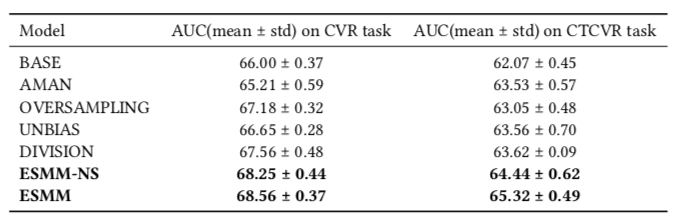• BASE: 普通建模 CVR 模型
• AMAN: 对负样本做 negative sampling
• OVERSAMPLING: 对正样本做 over sampling
• UNBIAS: 参考 这篇 paper 将 pCTR 当做 rejection probability
• DIVISION: 分别建模 pCTR 和 pCTCVR，然后利用上面的公式计算 pCVR
• ESMM-NS: 不 share bottom 的 ESMM
• ESMM: share bottom 的 ESMM

### IPS(Inverse Propensity Score)

Inverse Propensity Score 的做法是为每个有 label 的样本预估一个 propensity score(倾向性得分)，其含义直观来说就是样本进入训练集(被标记 label)的概率，如对于 CTR 模型，propensity 就是曝光的概率，对于 CVR 模型，propensity 就是点击的概率

IPS 实际上借鉴了 Importance Sampling 的思想，通过给每个样本一个概率值作为权重，从统计学上证明了基于观测到的数据求得的期望与全量数据的期望是一致，其推导过程可简单描述为如下方式

$$\min \sum_{i=1}^{L’} l(y_i, p_i) \tag{1}$$

\begin{align} Loss &= \sum_{i=1}^{L’} l(y_i, p_i)\notag \\ &= \sum_{i=1}^{L’} \frac{l(y_i, p_i)}{z_i} E_{O}(O_i)\notag \\ &= E_{O}(\sum_{i=1}^{L’} \frac{l(y_i, p_i)}{z_i} O_i)\notag \\ &= \sum_{i=1}^{L} \frac{l(y_i, p_i)}{z_i}\notag \end{align}

$$\min \sum_{i=1}^{L} \frac{l(y_i, p_i)}{z_i} \tag{2}$$

$$\min \sum_{i=1}^{L} (\frac{l(y_i, p_i)}{z_i} - l(\sigma_i, p_i)) + \sum_{i=1}^{L’} l(\sigma_i, p_i)$$

Domain Adatption 可以认为是 transfer learning 的一个子领域，根据 wiki 的说法其目标是

aim at learning from a source data distribution a well performing model on a different (but related) target data distribution.

1. Reweighting algorithms: reweight the source labeled sample such that it “looks like” the target sample (in terms of the error measure considered).
2. Iterative algorithms：iteratively “auto-labeling” the target examples
3. Search of a common representation space：construct a common representation space for the two domains
4. Hierarchical Bayesian Model：build a factorization model to derive domain-dependent latent representations allowing both domain-specific and globally shared latent factors

ESAM(Entire Space Adaption Modelling) 跟上面提到的 ESMM(Entire Space Multi-Task Model) 名字很相似，要解决的问题也很相似，但是前者是召回场景, 后者是 cvr 场景；

ESAM 总体如下所示，各符号含义如下（基本上就是向量化召回的涉及到的概念）

• $q$: query
• $d^s$: 有曝光的 item
• $d^t$: 没有曝光的 item
• $f_q$, $f_d$: 将 query 和 item 映射成 embedding 的函数
• $v_{q}$, $v_{d}$: query 和 item 被 $f_q$, $f_d$ 映射出来的 embedding
• $f_s$: 计算 $v_{q}$, $v_{d}$ 相似性的函数，常见的是內积
• $L_s$: 基于 $f_s$ 吐出的预估值 $S_{c_{q,d^s}}$ 计算的损失函数，常见的有 point-wise、pair-wise、list-wise 三大类
• $L_{DA}$、$L_{DC}^{c}$、$L_{DC}^{p}$: paper 中提出的缓解 exposure bias 的三种途径，以 loss 形式叠加在原始的 $L_s$ 上，也是下文要重点展开描述的部分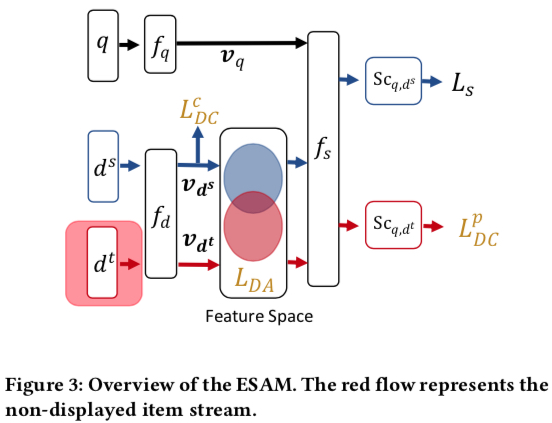ESAM 的核心在于叠加在原始损失函数 $L_s$ 上的三项：$L_{DA}$、$L_{DC}^{c}$、$L_{DC}^{p}$，下面会分别描述这三项的含义和计算方式

#### $L_{DA}$：Domain Adaptation with Attribute Correlation Alignment

(1) 相关性在 source domain 和 target domain 都是一致的
(2) 原始的特征(即 price、brand、material)会被映射到 embedding 中某一维或几维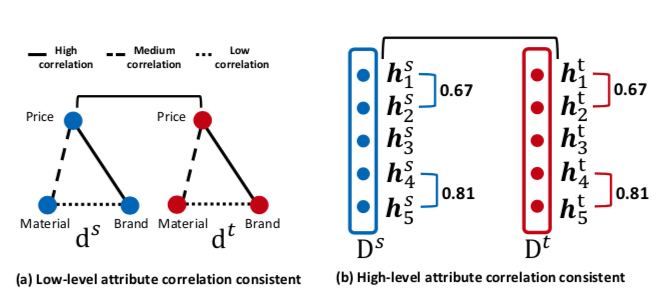$D^{s}=\lbrack h_{1}^{s},h_{2}^{s};\dots h_{L}^{s} \rbrack \in \mathbb{R}^{n×L}$
$D^{t}=\lbrack h_{1}^{t},h_{2}^{t};\dots h_{L}^{t} \rbrack \in \mathbb{R}^{n×L}$

\begin{align} L_{DA} &= \frac{1}{L^2}\sum_{(j,k)}({h_j^s}^T{h_k^s} - {h_j^t}^T{h_k^t})^2 \notag \\ &= \frac{1}{L^2} ||Cov(D^s) - Cov(D^t)||_F^2 \notag \end{align}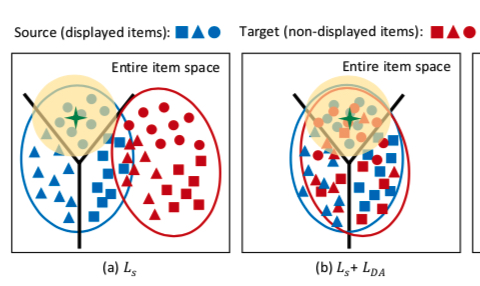#### $L_{DC}^{c}$：Center-Wise Clustering for Source Clustering.

\begin{align} L_{DC}^{c} &= \sum_{j=1}^{n} \max(0, ||\frac{v_{d_j^s}}{||v_{d_j^s}||} - c_{q}^{y_j^s}||_{2}^{2} - m_1) \notag \\ &+ \sum_{k=1}^{n_y} \sum_{u=k+1}^{n_y} \max(0, m2 - ||c_{q}^{k} - c_{q}^{u}||_{2}^{2})\notag \end{align}

$$c_q^k = \frac{\sum_{j=1}^{n} \delta(y_j^s = Y_k)\frac{v_{d_j^s}}{||v_{d_j^s}||}}{\sum_{j=1}^{n} \delta(y_j^s = Y_k)}$$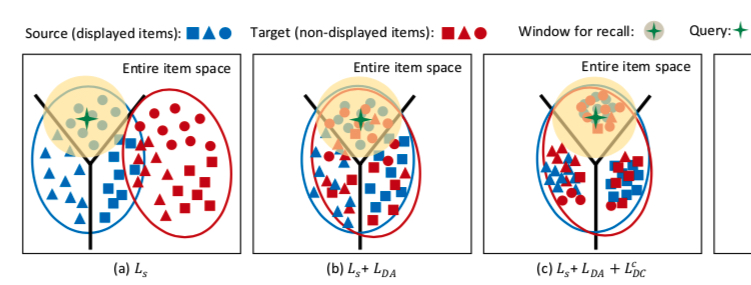#### $L_{DC}^{p}$：Self-Training for Target Clustering.

$$L_{DC}^{p} = -\frac{\sum_{j=1}^{n} \delta(S_{c_{q,d^t}} < p_1 | S_{c_{q,d^t}} > p_2)S_{c_{q,d^t}} \log S_{c_{q,d^t}}} {\sum_{j=1}^{n} \delta(S_{c_{q,d^t}} < p_1 | S_{c_{q,d^t}} > p_2)}$$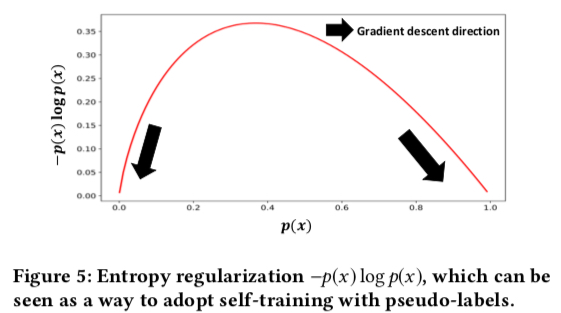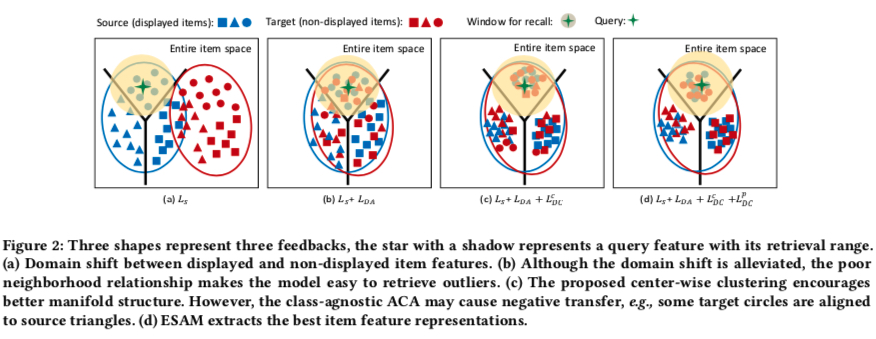$$L_{all} = L_s + \lambda_1 L_{DA} + \lambda_2 L_{DC}^{c} + \lambda_3 L_{DC}^{p}$$

## Summary

(1) Data Augmentation: 即利用那些 unlabeled 的样本，方法较多，如将所有样本都当做负样本、训练一个 imputation model 来给 unlabeled 的样本打上标签、通过 multitask 方式利用等
(2) IPS：只利用曝光的样本，从概率论推导出给曝光样本进行合适的加权后，基于曝光的样本求的期望是无偏的
(3) Domain Adaption：利用了 unlabeled 的样本，主要分析了 ESAM 这篇 paper, 同时通过在 loss 上添加了三项，能够令曝光和未曝光的 item 训练得到的向量空间尽可能保持一致，这三项的 loss 背后的思想也值得参考